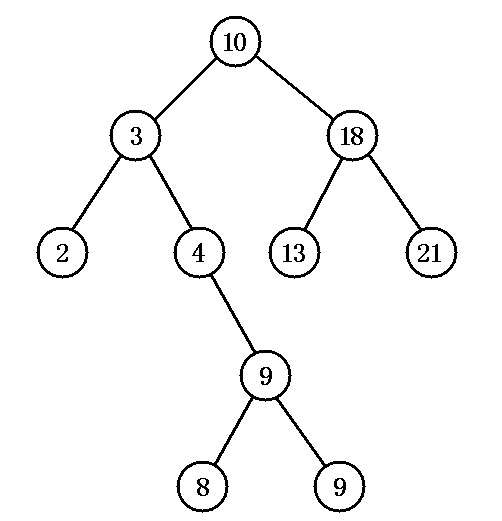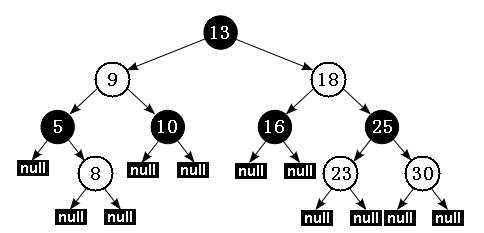红黑树

• 若它的左子树不空，则左子树上所有节点的值均小于它的根节点的值；

• 若它的右子树不空，则右子树上所有节点的值均大于它的根节点的值；

• 它的左、右子树也分别为排序二叉树。• 性质 1：每个节点要么是红色，要么是黑色。

• 性质 2：根节点永远是黑色的。

• 性质 3：所有的叶节点都是空节点（即 null），并且是黑色的。

• 性质 4：每个红色节点的两个子节点都是黑色。（从每个叶子到根的路径上不会有两个连续的红色节点）

• 性质 5：从任一节点到其子树中每个叶子节点的路径都包含相同数量的黑色节点。底层实现

实现的接口

public class TreeMap<K,V>
extends AbstractMap<K,V>
implements NavigableMap<K,V>, Cloneable, java.io.Serializable

public interface NavigableMap<K,V> extends SortedMap<K,V>

public interface SortedMap<K,V> extends Map<K,V>

• Comparable一般表示类的自然序，比如定义一个Student类，学号为默认排序；

• Comparator一般表示类在某种场合下的特殊分类，需要定制化排序。比如现在想按照Student类的age来排序。

• lowerEntry，返回所有比给定Map.Entry小的元素

• floorEntry，返回所有比给定Map.Entry小或相等的元素

• ceilingEntry，返回所有比给定Map.Entry大或相等的元素

• higherEntry，返回所有比给定Map.Entry大的元素

底层数据结构

static final class Entry<K,V> implements Map.Entry<K,V> {
K key;
V value;
Entry<K,V> left;
Entry<K,V> right;
Entry<K,V> parent;
//节点默认为黑色        boolean color = BLACK;
/** * 传入key，value，parent参数，创建新节点，子树为null，节点颜色默认为黑色。 */
Entry(K key, V value, Entry<K,V> parent) {
this.key = key;
this.value = value;
this.parent = parent;
}
/** * Returns the key. * * @return the key */
public K getKey() {
return key;
}
/** * Returns the value associated with the key. * * @return the value associated with the key */
public V getValue() {
return value;
}
/** * Replaces the value currently associated with the key with the given * value. * * @return the value associated with the key before this method was * called */
public V setValue(V value) {
V oldValue = this.value;
this.value = value;
return oldValue;
}
public boolean equals(Object o) {
if (!(o instanceof Map.Entry))
return false;
Map.Entry<?,?> e = (Map.Entry<?,?>)o;

return valEquals(key,e.getKey()) && valEquals(value,e.getValue());
}

public int hashCode() {
int keyHash = (key==null ? 0 : key.hashCode());
int valueHash = (value==null ? 0 : value.hashCode());
return keyHash ^ valueHash;
}

public String toString() {
return key + "=" + value;
}
}

//用来它定制排序规则，当它的值为null时，则使用key的自然顺序排序    private final Comparator<? super K> comparator;
//红黑树的根节点    private transient Entry<K,V> root;
/** * The number of entries in the tree */
private transient int size = 0;
/** * The number of structural modifications to the tree. */
private transient int modCount = 0;

重要方法

添加节点

put方法可以将一对key-value pair放到TreeMap中，当然也可以修改TreeMap中某个key对应的value值。在内部实现中，也需要将一个节点添加到红黑树中，这改变了原有红黑树的结构，因此需要做一些调整来保证修改后的树也符合红黑树的规则，让我们来看看源码中是怎么做的：

public V put(K key, V value) {
Entry<K,V> t = root;
if (t == null) {
compare(key, key); // type (and possibly null) check
root = new Entry<>(key, value, null);
size = 1;
modCount++;
return null;
}
int cmp;
Entry<K,V> parent;
// split comparator and comparable paths        Comparator<? super K> cpr = comparator;
if (cpr != null) {
do {
parent = t;
cmp = cpr.compare(key, t.key);
if (cmp < 0)
t = t.left;
else if (cmp > 0)
t = t.right;
else
return t.setValue(value);
} while (t != null);
}
else {
if (key == null)
throw new NullPointerException();
@SuppressWarnings("unchecked")
Comparable<? super K> k = (Comparable<? super K>) key;
do {
parent = t;
cmp = k.compareTo(t.key);
if (cmp < 0)
t = t.left;
else if (cmp > 0)
t = t.right;
else
return t.setValue(value);
} while (t != null);
}
Entry<K,V> e = new Entry<>(key, value, parent);
if (cmp < 0)
parent.left = e;
else
parent.right = e;
fixAfterInsertion(e);
size++;
modCount++;
return null;
}

put方法就是将新的Entry添加到二叉排序树上的过程，内容并不复杂，需要额外关注的是它调用的fixAfterInsertion(e)方法，该方法就是修复红黑树的过程，其源码如下，笔者已进行了详细地注释：

private void fixAfterInsertion(Entry<K,V> x) {
x.color = RED;
// 直到 x 节点的父节点不是根，且 x 的父节点不是红色        while (x != null && x != root && x.parent.color == RED) {
// 如果 x 的父节点是其父节点的左子节点            if (parentOf(x) == leftOf(parentOf(parentOf(x)))) {
// 获取 x 的父节点的兄弟节点                Entry<K,V> y = rightOf(parentOf(parentOf(x)));
// 如果 x 的父节点的兄弟节点是红色                if (colorOf(y) == RED) {
// 将 x 的父节点设为黑色                    setColor(parentOf(x), BLACK);
// 将 x 的父节点的兄弟节点设为黑色                    setColor(y, BLACK);
// 将 x 的父节点的父节点设为红色                    setColor(parentOf(parentOf(x)), RED);
x = parentOf(parentOf(x));
} else {// 如果 x 的父节点的兄弟节点是黑色                    // 如果 x 是其父节点的右子节点                    if (x == rightOf(parentOf(x))) {
// 将 x 的父节点设为 x                        x = parentOf(x);
rotateLeft(x);
}
// 把 x 的父节点设为黑色                    setColor(parentOf(x), BLACK);
// 把 x 的父节点的父节点设为红色                    setColor(parentOf(parentOf(x)), RED);
rotateRight(parentOf(parentOf(x)));
}
} else {// 如果 x 的父节点是其父节点的右子节点                // 获取 x 的父节点的兄弟节点                Entry<K,V> y = leftOf(parentOf(parentOf(x)));
// 如果 x 的父节点的兄弟节点是红色                if (colorOf(y) == RED) {
// 将 x 的父节点设为黑色                    setColor(parentOf(x), BLACK);
// 将 x 的父节点的兄弟节点设为黑色                    setColor(y, BLACK);
// 将 x 的父节点的父节点设为红色                    setColor(parentOf(parentOf(x)), RED);
// 将 x 设为 x 的父节点的节点                    x = parentOf(parentOf(x));
} else {// 如果 x 的父节点的兄弟节点是黑色                    // 如果 x 是其父节点的左子节点                    if (x == leftOf(parentOf(x))) {
// 将 x 的父节点设为 x
x = parentOf(x);
rotateRight(x);
}
// 把 x 的父节点设为黑色                    setColor(parentOf(x), BLACK);
// 把 x 的父节点的父节点设为红色                    setColor(parentOf(parentOf(x)), RED);
rotateLeft(parentOf(parentOf(x)));
}
}
}
// 将根节点设为黑色        root.color = BLACK;
}

删除节点

remove(key)方法就是从TreeMap中删除一对key-pair，也就是从红黑树中删除一个节点，进行该操作后也需要修复红黑树，具体代码如下：

public V remove(Object key) {
Entry<K,V> p = getEntry(key);
if (p == null)
return null;

V oldValue = p.value;
deleteEntry(p);
return oldValue;
}

private void deleteEntry(Entry<K,V> p) {
modCount++;
size--;

// If strictly internal, copy successor's element to p and then make p        // point to successor.        if (p.left != null && p.right != null) {
Entry<K,V> s = successor(p);
p.key = s.key;
p.value = s.value;
p = s;
} // p has 2 children
// Start fixup at replacement node, if it exists.        Entry<K,V> replacement = (p.left != null ? p.left : p.right);

if (replacement != null) {
// Link replacement to parent            replacement.parent = p.parent;
if (p.parent == null)
root = replacement;
else if (p == p.parent.left)
p.parent.left  = replacement;
else
p.parent.right = replacement;

// Null out links so they are OK to use by fixAfterDeletion.            p.left = p.right = p.parent = null;

// Fix replacement            if (p.color == BLACK)
fixAfterDeletion(replacement);
} else if (p.parent == null) { // return if we are the only node.            root = null;
} else { // No children. Use self as phantom replacement and unlink.            if (p.color == BLACK)
fixAfterDeletion(p);

if (p.parent != null) {
if (p == p.parent.left)
p.parent.left = null;
else if (p == p.parent.right)
p.parent.right = null;
p.parent = null;
}
}
}

// 删除节点后修复红黑树private void fixAfterDeletion(Entry<K,V> x) {
// 直到 x 不是根节点，且 x 的颜色是黑色   while (x != root && colorOf(x) == BLACK)
{
// 如果 x 是其父节点的左子节点       if (x == leftOf(parentOf(x)))
{
// 获取 x 节点的兄弟节点           Entry<K,V> sib = rightOf(parentOf(x));
// 如果 sib 节点是红色           if (colorOf(sib) == RED)
{
// 将 sib 节点设为黑色               setColor(sib, BLACK);
// 将 x 的父节点设为红色               setColor(parentOf(x), RED);
rotateLeft(parentOf(x));
// 再次将 sib 设为 x 的父节点的右子节点               sib = rightOf(parentOf(x));
}
// 如果 sib 的两个子节点都是黑色           if (colorOf(leftOf(sib)) == BLACK
&& colorOf(rightOf(sib)) == BLACK)
{
// 将 sib 设为红色               setColor(sib, RED);
// 让 x 等于 x 的父节点               x = parentOf(x);
}
else
{
// 如果 sib 的只有右子节点是黑色               if (colorOf(rightOf(sib)) == BLACK)
{
// 将 sib 的左子节点也设为黑色                   setColor(leftOf(sib), BLACK);
// 将 sib 设为红色                   setColor(sib, RED);
rotateRight(sib);
sib = rightOf(parentOf(x));
}
// 设置 sib 的颜色与 x 的父节点的颜色相同               setColor(sib, colorOf(parentOf(x)));
// 将 x 的父节点设为黑色               setColor(parentOf(x), BLACK);
// 将 sib 的右子节点设为黑色               setColor(rightOf(sib), BLACK);
rotateLeft(parentOf(x));
x = root;
}
}
// 如果 x 是其父节点的右子节点       else
{
// 获取 x 节点的兄弟节点           Entry<K,V> sib = leftOf(parentOf(x));
// 如果 sib 的颜色是红色           if (colorOf(sib) == RED)
{
// 将 sib 的颜色设为黑色               setColor(sib, BLACK);
// 将 sib 的父节点设为红色               setColor(parentOf(x), RED);
rotateRight(parentOf(x));
sib = leftOf(parentOf(x));
}
// 如果 sib 的两个子节点都是黑色           if (colorOf(rightOf(sib)) == BLACK
&& colorOf(leftOf(sib)) == BLACK)
{
// 将 sib 设为红色               setColor(sib, RED);
// 让 x 等于 x 的父节点               x = parentOf(x);
}
else
{
// 如果 sib 只有左子节点是黑色               if (colorOf(leftOf(sib)) == BLACK)
{
// 将 sib 的右子节点也设为黑色                   setColor(rightOf(sib), BLACK);
// 将 sib 设为红色                   setColor(sib, RED);
rotateLeft(sib);
sib = leftOf(parentOf(x));
}
// 将 sib 的颜色设为与 x 的父节点颜色相同               setColor(sib, colorOf(parentOf(x)));
// 将 x 的父节点设为黑色               setColor(parentOf(x), BLACK);
// 将 sib 的左子节点设为黑色               setColor(leftOf(sib), BLACK);
rotateRight(parentOf(x));
x = root;
}
}
}
setColor(x, BLACK); }

查询节点

get(key)方法是通过传入的key值来查找其对应的value，这一操作并不会改变红黑树的结构，源码如下：

public V get(Object key) {
// 根据指定 key 取出对应的 Entry
Entry>K,V< p = getEntry(key);
// 返回该 Entry 所包含的 value
return (p==null ? null : p.value); }

final Entry<K,V> getEntry(Object key) {
// 如果 comparator 不为 null，表明程序采用定制排序   if (comparator != null)
// 调用 getEntryUsingComparator 方法来取出对应的 key
return getEntryUsingComparator(key);
// 如果 key 形参的值为 null，抛出 NullPointerException 异常   if (key == null)
throw new NullPointerException();
// 将 key 强制类型转换为 Comparable 实例   Comparable<? super K> k = (Comparable<? super K>) key;
// 从树的根节点开始   Entry<K,V> p = root;
while (p != null)
{
// 拿 key 与当前节点的 key 进行比较       int cmp = k.compareTo(p.key);
// 如果 key 小于当前节点的 key，向“左子树”搜索       if (cmp < 0)
p = p.left;
// 如果 key 大于当前节点的 key，向“右子树”搜索       else if (cmp > 0)
p = p.right;
// 不大于、不小于，就是找到了目标 Entry
else
return p;
}
return null; }

final Entry<K,V> getEntryUsingComparator(Object key) {
K k = (K) key;
// 获取该 TreeMap 的 comparator
Comparator<? super K> cpr = comparator;
if (cpr != null)
{
// 从根节点开始       Entry<K,V> p = root;
while (p != null)
{
// 拿 key 与当前节点的 key 进行比较           int cmp = cpr.compare(k, p.key);
// 如果 key 小于当前节点的 key，向“左子树”搜索           if (cmp < 0)
p = p.left;
// 如果 key 大于当前节点的 key，向“右子树”搜索           else if (cmp > 0)
p = p.right;
// 不大于、不小于，就是找到了目标 Entry
else
return p;
}
}
return null; }

总结

TreeMap内部用红黑树保存数据，迭代顺序按照key值有序，与HashMap相比效率更低，只建议在需要按序索引key值时使用，它也是非线程安全的，key和value均不能为null值。